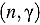The measurement facility QUANTOM is being developed for the material analysis of radioactive waste packed up in 200-L drums. QUANTOM enables a spatially resolved elemental analysis based on prompt gamma neutron activation analysis. The evaluation of the spatially resolved gamma spectra relies on the calculation of partialcross sections. Hereby, the neutron flux spectrum enters as a parameter, which needs to be simulated in the full three-dimensional geometry of the measurement facility. To ensure that the simulations can be carried out within an acceptable time frame, we use a deterministic neutron transport code specially developed for this purpose based on the SPN approximation of the linear Boltzmann equation. The following question arises: Does the approximation in the neutron transport model still allow a calculation of the partial cross sections at a sufficient level of accuracy. Therefore, in this paper, we study the calculation of partial cross sections in light of the approximation in the neutron transport model in the geometrical setting of the measurement facility. In a simulation study we consider four typical matrix materials and compare cross sections for all elements of the periodic table to reference results obtained by Monte Carlo simulations.